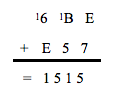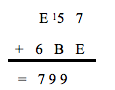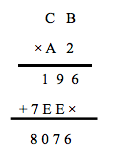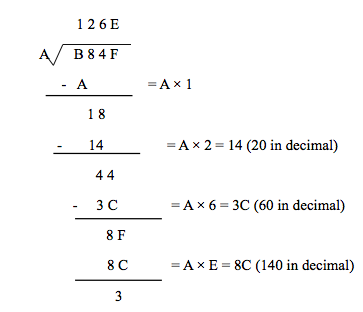## Hex Calculator

To use Hexadecimal Calculator, enter the values in the input boxes below and click on the Calculate button.

# Hex calculator

Hexadecimal addition is equivalent to the addition of decimal numbers. The addition of the letters A, B, C, D, E, and F is the only difference. When values exceed 9, it could be easier to convert from the hexadecimal system to the decimal one. Here is an example of hex addition.E + 7 in this case is represented by the number 14 + 7 = 21. The hex equivalent of 21 in decimal is 15. The 1 moves to the following column in the same manner as the decimal addition. The subsequent column equals 1 + B (11) + 5 = 17 in decimal and 11 in hex, respectively. Add 1 to the last column, which gives the result 1 + 6 + E (14), which is 21 in decimal and 14 in hex. This results in 1515 in hex.

## Hex Subtraction

The process for computing hex subtraction is the same as that for computing decimal subtraction, with the main distinction being that when borrowing in hex, the "1" borrowed represents 16decimal rather than 10decimal. This is due to the borrowed column's hex size being 16 times greater than its decimal size of 10. The hex subtraction example is shown below.In the first column, E, or 15 in decimal form, is smaller than 7, which. Thus, we must borrow from the following column. This reduces the five to four and loans one, or 16 decimal, to the first column, making the result 16 decimal + seven decimal - E, or 14 in decimal, equal to nine. 4 is now smaller than B in the second column (11). We must once more borrow from the following column. This changes the E to D and adds 1 or 16 decimal places to the second column, making the result 9 (16 decimal + 4 minus B or 11 decimal places). The computations are straightforward because the last column doesn't require any borrowing: D, or 13 in decimal form, - 6 = 7, giving the value 799.

## Hex Multiplication

Due to the bigger conversions between hex and decimal, hex multiplication can be challenging. Here is a hex multiplication example.Multiplication steps for each number is shown below

2 × B (11 in decimal) = 22 that is 16 in hex

2 × C (12 in decimal) = 24 that is 18 in hex

A (10 in decimal) × B (11 in decimal) = 110 that is 6E in hex

A (10 in decimal) × C (12 in decimal) = 120 that is 7E in hex

## Hex Division

Hexadecimal division is the same as decimal division, with the exception that we must convert from hex to decimal, carry out long division in decimal, and then convert back to hex after we are finished. Below is an example of hex division.## How to Use the Hex Calculator?

The hex calculator should be used as follows:

Step 1: Enter two hexadecimal numbers in the input field

Step 2: To obtain the conversion, click the "Convert to Decimal & Binary" button.

Step 3: In the output field, the hexadecimal number will finally be converted to a decimal and binary number with its arithmetic operations.

## ToolBox Hex Calculator

To perform hex addition, hex subtraction, hex multiplication, and hex division without a pen and paper, use a hexadecimal calculator. An efficient tool for adding two hex values is the hex adder calculator. Similar to this, a hex calculator can quickly multiply, divide, subtract, and add two provided integers using four separate algorithms. If you'd rather convert numbers to hex than calculate them, you can use a hex to binary converter or a binary to hex converter.

Recent Blog Posts